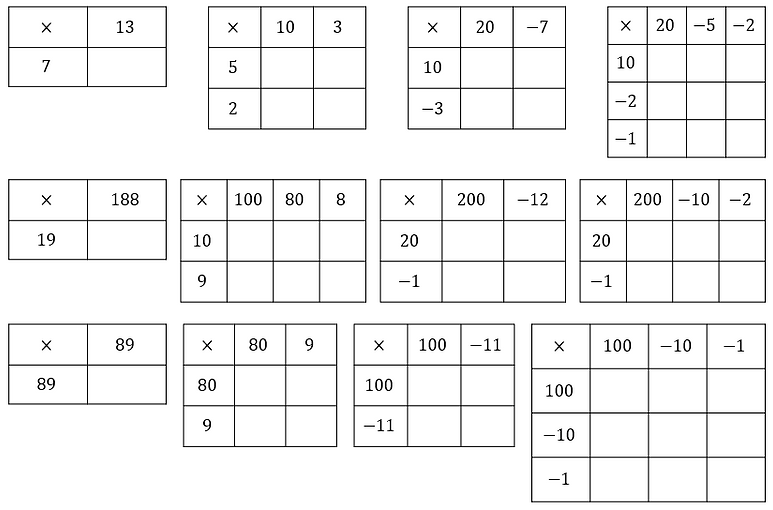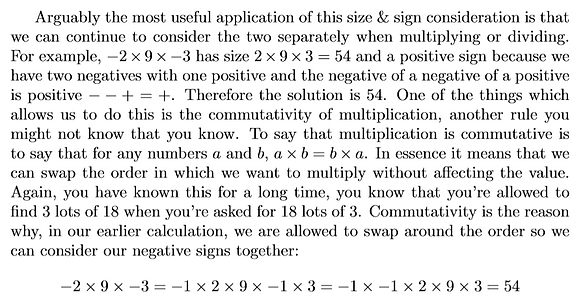top of page## Negative Behaviour

The negative numbers are simple but troublesome. It's easy enough to appreciate that they must exist, mathematics cannot break just because we want to take larger from smaller. Some of their behaviour is logical too: the sum of two negatives should be more negative, it feels right, and it is. Even subtracting a negative can be reasoned around in our heads, if you take away a bad thing you feel better, so subtracting a negative should take us in the positive direction, which it does. The kicker though is multiplication (and subsequently division). There is no real example we can use to illustrate it 'If Ciara has -3 packets of apples and each packet conatins -8 apples how many apples does she have?' because, though the negative numbers exist, we can't really see them out in the world unless we put them there, to measure temperature below freezing or depth below sea level for instance. There is no real reason why negative lots of a negative thing should somehow result in a positive thing. But that's okay, maybe this isn't a should: this is a must. Mathematics is art but it's art that's got rules and when anything new comes along it is bound to comply with those rules, it cannot disturb the peace. The following exercises should sharpen your skills when it comes to all of the negatives' behaviour and help you get a sense of why they play the way they play; because they must.

## A Rule You Don't Know You KnowWe can also observe the distributivity of multiplication over addition in the most basic real life interpretation of multiplication as 'lots of'. If we want to serve four lots of strawberries and ice cream we may well serve four lots of strawberries first and then add four lots of ice cream, the outcome is the same. Similarly, if we very generously give three friends £5, we can hand out 3 lots of £5 or (provided we have the change) we can hand out 3 lots of £1 and repeat the process five times. Again, the outcome is the same, it simply must be.

It is the real world that tells us this distributive law must be true, we can see it working. The trick is that once we've discovered the law in the things we can see, it can help us make sense of the things that we can't.

## Obeying Distributivity## You Can Distribute Too

Try the expansions below to feel distributivity working over different kinds of numbers. You can come up with your own examples too, see how far you can push the rule, it won't break.## Size & Sign

Another way to look at the integers is to consider the symmetry of the set. Zero sits in the middle with the natural numbers queueing infinitely upwards and their negative partners mirroring them exactly on the other side of zero. This way of thinking leads us to the understanding that any natural number, say 7, and its negative, -7, are the same distance from the mirror line at zero. Consequently, 7 and -7 have exactly the same size (also referred to as magnitude from the Latin for greatness/size) since the size of a number is measured by distance from zero. This in turn leads us to the counterintuitive circumstance in which -10 is simultaneously bigger and less than -7. We might need to lay on the ground a while to think about this but eventually it makes sense if we hang on to the idea of size and sign.

Size

Zero is nothing and so has no size. Size is a non-negative quantity, in that there can be no size smaller than no size. Therefore, to move away from zero in any direction is to get bigger. Any number that's not zero, negative or otherwise, must be bigger than zero. The size of a number is measured by how far it is away from no size, from zero. 90 is bigger than 20 because 90 is further from 0. Similarly, -18 is bigger than -12 because -18 is further from 0. Again similarly but somewhat more surprisingly, -50 is bigger than 49 because -50 is further from 0. Reassuringly, 49 remains more than -50 because 49 is higher on the numberline. To decide which number is greater or lesser than another we still look to their positions on the numberline, the higher is greater, the lower is lesser. This means that below zero, smaller negatives are closer to zero and therefore are 'greater than' bigger negatives which are further away. Hence the peculiar at first but perfectly true statement '-10 is simultaneously bigger than and less than -7'. If it still doesn't feel right draw a numberline vertically about zero on a wall, lay on the floor and stare at it for a while, you'll get there.

Sign

Size tells us the distance from zero, so 7 and -7 are both a distance of seven from zero. The sign therefore distinguishes between the two by telling us which side of that mirror line we're on, the positive above zero or the negative below. Thus we can think of 'taking the negative' as a reflection. If we begin at 19 and take the negative, we reflect in the mirror line at zero and arrive at -19, notably size is unchanged. If we then take the negative again we must reflect again, which in effect is reflecting back to where we started, leading to the fact that -(-19)=--19=19. This gives us another way of understanding that negative multiplied by negative must be positive because the negative 'of' a negative is positive. We can carry on taking negatives as long as we wish, -------19=-19 and ------------19=19. Although it is not clear that this particular example is deeply useful there are some very practical applications for the reflective property of taking the negative.

## Another Rule You Don't Know You KnowIt is commutativity that explains the deeply pleasing fact that 8% of 25 is 25% of 8. If you haven't seen this before it's a real treat, see commutativity at work in the swap in the middle.

Here are some other nice examples to enjoy at home/impress with on first dates.

## That Negative OneSuppose we want to calculate:

If we know enough about -1 then we can simplify the left fraction all the way and the subtraction becomes very simple:

However, if we don't know how to manipulate algebraic fractions using -1, then we might have got stuck at the penultimate stage of the simplification on the left, in which case we must work much harder to perform the subtraction:

As you can see, it pays to know how -1 plays.## Get Negative

Each column can be separated into two distinct groups of equivalent fractions. For those involving quadratics it is useful to factorise and observe how the factors in brackets can change together without affecting the value of the fraction.bottom of page